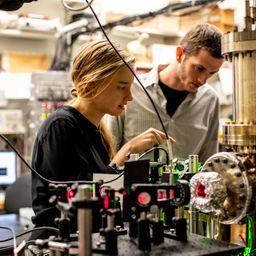## no answer available

Quantum Physics

Atomic Physics

### Discussion

You must be signed in to discuss.
##### Top Physics 103 EducatorsLB##### Jared E.

University of Winnipeg### Video Transcript

and this problem. We're told that light falls on a sample of silver and then we need to 0.5 volts to stop of the photons, so the stopping voltage is equal to 2.5 bolts. And so, um, we want to get the max kinetic energy. So, um, basically, for that, we want to do Eve the kinetic energy. It's stopped by the stopping potential. So you cannot account of the potential Energy comes from the kinetic energy. So you want to set these equal, And then if you take this in, multiplied by E, that's the same thing is converting a number two electron volts. So then this is going to be 2.5, you hear? And then for B, we want to get the maximum speed of the, um, ejected photo electrons. And so K is equal to 1/2 and b squared. And then we get B. Is he cool, too? The square root of two K e over AM and Katie. We need to convert that to jewels, so we'll just write it down just to be totally clear. So it's gonna be 25 evey times 1.6 times 10 to the minus 19 Jules per e v and so multiplying that out E 1.6 making well, I'll just go ahead and copulate everything, actually. So these courage teen times two times a kinetic energy divided by the matter. Me, the massive intellectual in the mass of the electron is 9.11 times 10 to the minus 31. And with that we got the numbers. It's a really high speed. We get, um three, 9.4 times 10 of the fifth meters per second. So it's quite fast and you want to get the wavelength of the incident light. And so what we want to do. ISS Let's see, um, we wanted So we want to get the wavelength of incident light. So we want to use this formula for that. So the main formula for the photoelectric effect is kinetic. Energy is the energy of the proton, Mom. So I'll call that BP and then not plus minus the work function. So we know the cosmic energy and then we want to got the from that's that's this quantity and I just use he be. And then we know the work function that which tells us. So we want to get the energy of the photons and then from back of the, um, wavelength and then in general for that it the energy of a photons agency over Lambda. So, um, this equation becomes this and so you can get that lum kind of rearranging it. Bring fire to the other side. So five plus k e um, And then that's equal to HC over lambda. And then if you cross multiply, you get that lambda is HC divided by five plus K e. And this is what I use for K E. And then for Fei gives it to us in the problem. So, um, go ahead and calculate this. So that's 6.626 times kind of the miners 34 times 2.9 any past him to the all right by the work functions. And then I'm gonna have to convert those work function and the the stopping bolt interested to 2.5 plus 4.73 and then rose on TV. Everything else is an s. I so I need to multiply them by 1.62 friends 10 to the minus 19 and the of Attica 172 nano metersUniversity of Washington

#### Topics

Quantum Physics

Atomic Physics

##### Top Physics 103 EducatorsLB##### Jared E.

University of Winnipeg